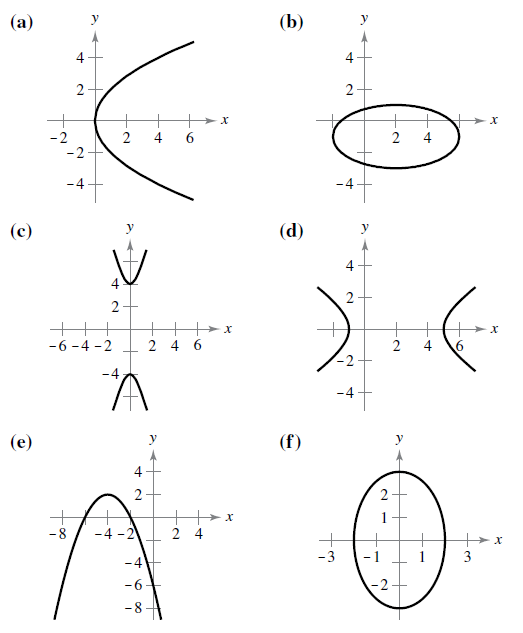Chapter 10.1, Problem 7E

Chapter
Section
Textbook Problem

Matching In Exercises 5-10, match the equation with its graph. [The graphs are labeled (a), (b), (c), (d), (e). and (f).]y 2 16 − x 2 1 = 1

To determine
The correct match of the equation y216x2=1 with its corresponding graph.

Explanation

Given: The equation y216x2=1 and the graph of equations are,

Calculation: Consider the equation y216x2=1. Since the given equation is of the type x2a2y2b2=1 which is the standard form of the hyperbola and the hyperbola has a center at (h,k) that (0,0) with semi axis a=4 and semi conjugate axis b=1

Still sussing out bartleby?

Check out a sample textbook solution.

See a sample solution

The Solution to Your Study Problems

Bartleby provides explanations to thousands of textbook problems written by our experts, many with advanced degrees!

Get Started

Evaluate the expression sin Exercises 116. (23)2

Finite Mathematics and Applied Calculus (MindTap Course List)

6x2(3x2+2)+14(x+2)

Applied Calculus for the Managerial, Life, and Social Sciences: A Brief Approach

Proof Prove that if f has an inverse function, then (f1)1=f

Calculus: Early Transcendental Functions (MindTap Course List)